## Artigo

•Citado por SciELO
•Acessos

•Citado por Google
•Similares em SciELO
•Similares em Google

## versão impressa ISSN 0120-6230

### Rev.fac.ing.univ. Antioquia  no.78 Medellín mar. 2016

#### http://dx.doi.org/10.17533/udea.redin.n78a02

ARTÍCULO ORIGINAL

A matheuristic algorithm for the three-dimensional loading capacitated vehicle routing problem (3L-CVRP)

Un algoritmo híbrido para el problema de ruteo de vehículos con restricciones de carga de tridimensional

Luis Miguel Escobar-Falcón1*, David Álvarez-Martínez2, Mauricio Granada-Echeverri1, John Willmer Escobar3, Rubén Augusto Romero-Lázaro2

1Facultad de Ingenierías, Universidad Tecnológica de Pereira. Carrera 27 #10-02 Barrio Álamos. A. A. 097. Pereira, Risaralda, Colombia.

2Departamento de Ingeniería Eléctrica, Universidad del Estado de São Paulo. Campus de Ilha Solteira, Av. Professor José Carlos Rossi, 1370-Campus III. CEP: 15385-000. Ilha Solteira, Brasil.

3Departamento de Contabilidad y Finanzas, Facultad de Ciencias de la Administración, Universidad del Valle. Calle 4B # 36-00, Sede San Fernando, Edificio 124. A. A. 25360. Cali, Colombia.

* Corresponding author: Luis Miguel Escobar Falcón, e-mail: luismescobarf@gmail.com

(Received December 2, 2014; accepted January 29, 2016)

ABSTRACT

This paper presents a hybrid algorithm for solving the Capacitated Vehicle Routing Problem with practical three-dimensional loading constraint. This problem is known as 3L-CVRP (Three-dimensional Loading Capacitated Vehicle Routing Problem). The proposed methodology consists of two phases. The first phase uses an optimization procedure based on cuts to obtain solutions for the well-known Capacitated Vehicle Routing Problem (CVRP). The second phase validates the results of the first phase of a GRASP algorithm (Greedy Randomized Adaptive Search Procedure). In particular, the GRASP approach evaluates the packing constraints for each performed route of the CVRP. The proposed hybrid algorithm uses a relaxation of the classical model of two sub-indices for the vehicle routing problem. Specifically different types of cuts are added: subtour elimination, capacity-cut constraints, and packing-cut constrains. The proposed algorithm is compared with the most efficient approaches for the 3L-CVRP on the set of benchmark instances considered in the literature. The computational results indicate that the proposed approach is able to obtain good solutions, improving some of the best-known solutions from the literature.

Keywords: Vehicle Routing Problem, Matheuristics, Branch-and-Cut, packing

RESUMEN

Palabras clave: Problema de enrutamiento de vehículos, Matheurísticas, Branch-and-Cut, empaquetamiento

1. Introduction

Many actions in the transit of products involve two problems, which have been studied deeply in the last decades. The first problem is referred to the design of the routes to fulfill the demand of the customers by considering the minimum travelling cost (Capacitated Vehicle Routing Problem-CVRP). The second problem considers the best way to load the products in the used vehicles for the performed routes (Three-dimensional Container Loading Problem – 3D-SLOPP). These problems belong to the well-known NP-hard problems for which the solution is really challenging.

The Vehicle Routing Problem (VRP) arises in the distribution of a set of products to a number of customers who are geographically dispersed, by regarding the minimization of the distribution costs or the maximization of the net income associated with the transportation. The Three-Dimensional Container Loading Problem or Three-Dimensional Knapsack problem seeks to accommodate a number of elements within a rectangular box (container) by considering several objective functions and by satisfying determined packing constraints. The combination of both problems has several realistic applications in many industrial contexts such as the transportation of chipboard for furniture, the delivery of courier companies, the conveyance of vehicles, and the transit of products on pallets, among others.

The combined problem of routing and packing considered is a variant of the well-known routing problem called Capacitated Vehicle Routing Problem (CVRP) and the variant of the packing problem called Container Loading Problem (3D-SLOPP). The CVRP seeks to perform a set of routes starting and ending at a central depot. The CVRP could be defined as a set of Khomogeneous vehicles (each one having a capacity Q ), which must satisfy the demand of a set of N customers. Each vehicle is assigned to at least one route. A single vehicle must visit each customer one time. The sum of the demands of the customer visited on a single route must not exceed the vehicle capacity Q . The objective is to minimize the sum of the traveling cost for the performed routes.

The 3D-SLOPP must be solved for each performed route. The 3D-SLOPP consists in loading a set of small boxes B inside a container. The set Bhas different sizes and limited amounts. The objective is the maximization of the available occupied space. This problem is also well known as the three-dimensional knapsack problem (3D-SKP) or Three-Dimensional Single Large Object Packing Problem (3D-SLOPP). For the 3D-SLOPP, the orientation and the fragility of the boxes, the load stability, and the sequence of the load must be considered. Several variants of the 3D-SLOPP are obtained by considering different type of packing constraints. In this work, we also study the variant of the 3D-SLOPP problems by taking into account that the vehicle capacity Qis equal to its volume, which is attractive for many real applications of the industry. The integration of both problems arises to the well-known problem 3L-CVRP, i.e. Three-dimensional Loading Capacitated Vehicle Routing Problem.

In this work, we have proposed a matheuristic algorithm, which is computationally compared with the most effective heuristics for the 3L-CVRP. The new proposed approach obtains competitive results on the classical set of benchmarking instances for the 3L-CVRP. The main contribution of this paper is to propose a new successful matheuristic approach for the 3L-CVRP by considering a combination of exact techniques with a GRASP approach which guarantees the loading constraints. The proposed algorithm is a novel matheuristic approach which combines a GRASP approach with exact algorithm for getting good results. While a combination between exact techniques and Tabu Search (TS) has been proposed in the literature for the CVRP (see e.g. ) no attempt has been proposed for combining exact techniques with a GRASP scheme for the 3L-CVRP. The former algorithm is able to improve the best-known solutions found by the most effective published algorithms on a set of instances taken from the literature.

The paper is organized as follows. The literature associated to the Capacitated Vehicle Routing Problem and the Packing Problem is described in Section II. Section III gives a formal definition of both problems into the 3L-CVRP and the literature proposed to solve it. Section IV presents a detailed description of the framework used by the proposed algorithm. A computational comparative study on the classical set of benchmark instances from the literature is provided in Section V. Finally, Section VI contains concluding remarks and future research.

2. Literature review

The vehicle routing problem considering load constraints is a relative new interesting research subject. The interest of researchers and practitioners is motivated by the intrinsic difficulty in this area, which combines two NP-hard problems: the Capacitated Vehicle Routing Problem and the Container Loading Problem.

2.1. Capacitated Vehicle Routing Problem (CVRP)

The Capacitated Vehicle Routing Problem (CVRP) seeks to find a specified number of cycles (routes) to fulfill the demand of a set of vertex (customers) by starting and finishing at a central depot located in the vertex 0. A complete formulation of the CVRP proposed in , which is well known as formulation of two indices is shown as follows:

The CVRP could be described as graph theory problem. Let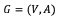be a complete graph, where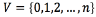is the vertex set and Ais the arc set. Verticescorrespond to the customers, whereas vertex 0 corresponds to the depot. A non-negative traveling cost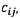is associated with each arc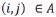. The traveling cost between (j, i) is not allowed. Therefore, the cost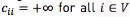. In particular, this paper considers the symmetric version of the CVRP (SCVRP). Therefore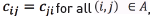and the arc set could be replaced by a complete set of undirected edges, E . Each vertex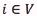is associated with a known nonnegative demand,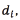to be delivered. Note that the depot has a fictitious demand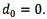Given an edge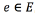, let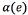and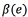denote its endpoint vertices. Given a vertex set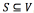, let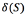and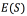denote the set of edges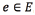that have only one or both endpoints in S , respectively. In addition, let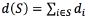be the total demand of the set S.

A set of Kidentical vehicles, each with capacity Q , is available at the depot. To ensure feasibility we assume that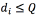for each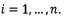Each vehicle performs only one route.For a set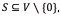we denote by r(S) the minimum number of vehicles needed to serve all customers in S. Often, r(s) is replaced by the trivial Bin Packing Problem lower bound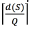. The BPP allows determining the minimum number of bins (vehicles), each one with capacity Q, required to load all the n items, each with nonnegative weight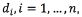being NP-hard in the strong sense.

The CVRP consists of finding a set of Kperformed routes (each one corresponding to one vehicle) with minimum cost, defined as the sum of the costs of the arcs belonging to the performed routes. The CVRP is subject to the following constraints

i.      Each route ends and begins in the depot vertex

ii.     Each customer vertex is visited by exactly once; and

iii.    The sum of the demands of the vertices visited by a route must not exceed the vehicle capacity, Q .

The model employed in this paper, is a two-index vehicle flow formulation that uses binary variables x to indicate if a vehicle travels an arc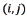in the optimal solution (7). In other words, variable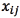takes value 1 if arc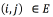belongs to the optimal solution and takes value 0 otherwise. The objective function is to minimize the cost of the traveled arcs (1). The Eqs. (2-6) control the visits to the clients and the subtour elimination.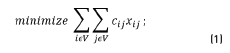Subject to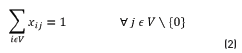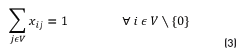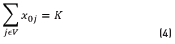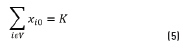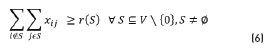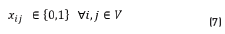Constraints (2) and (3) correspond to the flow constraints of the set V(customers) i.e., these constraints guarantee that each customer must be visited by one single vehicle only once. Constraints (4) and (5) ensure that the same number of routes arrive and leave the depot 0. Eq. (6) consider the subtour elimination constraints. According to , this set of constraints could be interpreted as follows in the Eq. (8):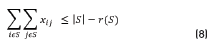However, the set of subtour elimination constraints require special considerations due to its combinatorial complexity when the number of customers is increased. Therefore, we have considered the set of constraints (8) for the proposed algorithm. In particular, we added the required cuts to eliminate subtour until a feasible solution is found during the branch and bound procedure.

Note that the considered model is able to represent only the CVRP problem. For getting a global representation of the 3L-CVRP problem, we have considered the set of constraints (9), which are added iteratively together with the subtour elimination constrains. This set of constraints guarantee the feasibility of the packing requirements (such as multi-drop constraints, among others). Let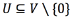be a subset of customers with a cumulative demand, which cannot be packed in the vehicles by considering the sequential packing or multi-drop constraints. All the possible subsets of customers who can not be packed, are controlled by constraints (9):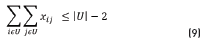By adding this set of constraints, the mathematical model could represent properly the 3L-CVRP problem. The level of difficulty of the solution of the model (1) – (9) increases when the number of nodes increases, due to the combinatorial explosion of possible subsets of customers controlled by Eq. (8). The high complexity of the CVRP has led the development of the various algorithms on exact and approximate methods. The CVRP has been investigated since the decade of the 50's. Reviews of the CVRP are presented in [2-4]. In , a vehicle routing problem arising in supply chain management is proposed (including the 3L-CVRP).

In realistic loading and packing problems, the demand of the customers is not simply characterized by a quantity (as in the case of CVRP), but it also is determined by its shape and location in the space. In this case, it is necessary to ensure that an item to be carried on must be placed into the space used by a vehicle. These constraints are concerned with the multidimensional rectangular packing problems, which originate as an extension of the one-dimensional Bin Packing Problem (BPP). The BPP can be described as the problem of placement of a set of segments without overlapping. A general introduction to the rectangular packing research area is given by [6-9].

3. Problem definition

The 3L-CVRP considers that three-dimensional items generate the full weight of demand of customer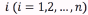. Each item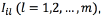has a width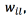a high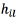and a length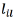. The loading surface of each vehicle has a width W, a high H, and a length L. Let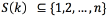, be the set of customers visited by the vehicle K. The 3L-CVRP imposes a packing constraint without any variety of three-dimensional overlap of all items ordered by each customer S(K) within the cargo space of dimensions WxHxL. The packing constraints for the 3L-CVRP are characterized by the following aspects:

· Orientation: Items have a fixed orientation or can be rotated 90 º in the horizontal plane by keeping off the rotation of the vertical orientation.

· Fragility: An item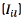could have a fragility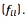If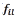is equal to 1,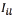is fragile, and 0 otherwise. In this case, non-fragile items cannot be placed over fragile items.

· Area support: Each itemis packaged over other items. Let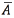be the area of the bottom of product. The packaging is feasible only if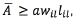where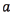is a given threshold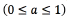and represents the minimum portion of the area of the box, which is contacted with box of item(item for which the current box is supported).

· Sequential load: When an item is unloaded, there must be a chronological succession of straight movements in the direction of the rear of the vehicle, allowing the process of unloading without moving any other item. In other words, any item requested after customer i may be placed onor betweenand the backside of the vehicle.

This work considers all the packing constraints by characterizing them as previously expressed. It is worth to note that this characterization considers many assumptions limiting the functionality and applicability on a real context. For example, the constraint of fragility could be formulated as a binary expression depending of the load-bearing strength and the orientation of the boxes .

The previous published works for the 3L-CVRP have tried to eliminate some of the packing constraints in order to distinguish the most critical. In , the authors indicate that the loading sequence is usually the dominant constraint. In this work, other features of the problem are studied; particularly, the fact of the vehicle capacity Q is usually specified as a parameter without any relation to the type of load. Therefore, this aspect implies that the value of d (i) (demand for each customer) assumes that all the boxes for the set of customers have the same density of material with different demand; i.e. each customer has different types of boxes with different density but all the boxes must have the same density.

There are two type of assumptions respect to the vehicle capacity constraints:

· Transportation of all the type of material density of boxes for each customer

· Transportation of one type of material density of the boxes (which is assumed as 1, being the weight of each box equal to its volume).

The previous published works only consider the first assumption. We have considered both assumptions (see results of Matheuristic column and Matheuristic (3L-VRP) column in Table 1). Indeed, one contribution of the proposed work is to examine the effect of the density equal to 1. Consequently, for the second assumption, the new value of d(s)will be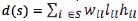and the new vehicle capacity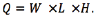All the set of packing constraints are modeled and linked with the transportation model in , but due to the high complexity of the model, the proposed methodology is inadequate for solving medium and large size problems. In this work, a GRASP algorithm within the mathematical model guarantees these constraints, allowing the solution of real problems of the companies. Several approximate algorithms have been proposed to solve the 3L-CVRP.

The problem of 3L-CVRP by considering time window constraints is proposed in . In this work, several constructive heuristics are addressed. In , an extension of the previous described work () is presented. In particular, a multi objective scheme is proposed by considering the following objective functions: minimizing the number of vehicles, minimizing the total travel distance, and maximizing the used volume.

In , a Tabu Search algorithm is proposed for solving the 3L-CVRP. In this work, for each neighborhood solution of the vehicle routing problem, the payload is determined by another Tabu Search scheme for the Three-dimensional Strip Packing Problem (3SPP). If the resulting load exceeds the capacity of the vehicle, the solution is accepted by considering a penalization scheme. Other Tabu Search algorithm has been proposed by . The loading problem is solved by a heuristic for the minimal waste of space. Finally, a tabu search algorithm solves the corresponding routing problem.  propose a generalization of the three-dimensional for the bi-dimensional case. In , an Ant Colony Optimization (ACO) is presented for the 3L-CVRP. Heuristic approaches for which the vehicle routing problem is solved by metaheuristic algorithms based on population are given in [19, 20]. In , a bee metaheuristic algorithm with a Tabu Search is proposed for the 3L-CVRP. A heuristic algorithm for the loading problem with a genetic algorithm for the 3L-CVRP has been proposed in . In , two heuristics for the packing problem are improved and introduced within a Tabu search scheme for solving the considered problem. Experiments computational show the efficiency of the proposed approach.

An uncapacitated 3L-CVRP is introduced in . Two heuristic approaches to solve this variant of the original problem are proposed and compared. A two-stage heuristic for solving the problem considered in  is presented in . The first stage optimizes the packing problem, while the second deals with the aspect of the corresponding routing problem. Computational experiments show the high efficiency of the method.

In , the author introduces an efficient hybrid approach based on a Tabu search algorithm for the vehicle routing subproblem. In the proposed approach, the generated routes are ordered in a list, which is sorted increasingly according to the travelling cost. For each solution in the resulting list, a tree search algorithm for solving the loading subproblem is performed. Computational experiments show the effectiveness of the proposed methodology.

Note that all the proposed approaches proposed for the 3L-CVRP are based on heuristic schemes except for . In this paper, we propose a matheuristic algorithm, which differs from  because the routing problem is solved by an exact method and the packing problem by an approximate algorithm. The proposed algorithm is explained in the following sections.

4. Matheuristic approach

The general solution strategy proposed addresses both problems separately: the Capacitated Vehicle Routing Problem (CVRP) and the Three Dimensional Container Loading Problem (3D-SLOPP). In particular, for each solution of a CVRP, a validation of the packing constraints of the cargo of the containers for each route is performed. The main strength of the proposed approach is that the computational effort is mainly focused on the exact solution for the CVRP, while the loading problem is solved by a GRASP approach of high performance. The GRASP approach is calibrated according to the characteristics of the items to be delivered, i.e., the cumulative demand of the customers covered by each route.

The mathematical model of two-index (1-9) is relaxed by eliminating the capability and the subtour elimination constraints. The proposed approach gradually inserts these constraints during the branch and cut scheme in order to obtain feasible solutions. Indeed, the proposed algorithm begins with an initial solution generated by the well-known Clark & Wright algorithm and validated by the GRASP algorithm. The objective function value of the initial solution is used as upper bound of the proposed approach.

The algorithm allows infeasible solutions for the 3L-CVRP due to that the first feasible solution found during the search corresponds to the optimum of the problem. In particular, when a feasible solution for the routing problem is found, it provides a lower bound for the original problem. Therefore, it can be used as initialization for the 3L-CVRP during the search. The upper bound is given by the corresponding load demand for the Kperformed routes (the problem is studied as a bin-packing). However, the effort of the proposed algorithm is totally oriented to find feasible solutions for the vehicle routing problem guided by the minimization of total travelling cost. Although the two-index model (1-9) requires a notable computational effort, it allows articulating properly the constraints related with the management of the loading of the boxes in the container.

The 3L-CVRP has been addressed by applying an exact method (branch-and-cut) for the vehicle routing problem solved by ILOG Concert Technology and CPLEX. The packing problem is solved by a GRASP approach. Initially, the relaxed version of the model (1-9) is solved without the capacity and elimination of subtour constraints. Then, these constraints are added iteratively to the branch and cut process together with the packing constraints during the search procedure. The algorithm finishes when a solution is found for the model involving all the constraints. The matheuristic algorithm is described as follows:

4.1. Exact method for the Vehicle Routing Problem

In the proposed algorithm, we have considered the two-index model (1-9) to solve the subproblem of CVRP. This formulation is based on subsets of Scustomers to control the appearance of subtours and the capacity constraints of the vehicles. Constraints (6) or (8) are eliminated obtaining a relaxed model (MCVRP).

In particular, the proposed approach starts by solving the MCVRPand by keeping its optimal solution in S. Then, the current optimal solution for the MCVRPis checked to find violations of capacity constraints (Lu) or the presence of subtours (Ls). If this solution is infeasible, the corresponding cuts are added to avoid infeasibilities by the function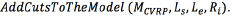If the solution is feasible, the procedure must check the packing of demand for each route by a GRASP approach, which determines whether it is possible to pack the boxes into the vehicles with a pattern of loading without rearrangement. In addition, if the inverse route (inverse position of the customers) is impossible to pack, the considered routes must be prohibited (Lu). The pseudocode of the proposed algorithm is detailed in Figure 1.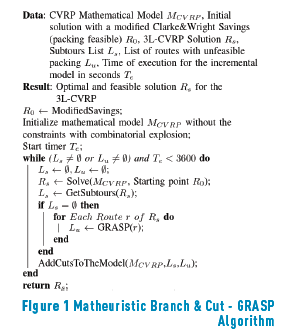In order to avoid the appearance of routes violating the packing constraints, the cuts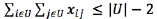(9) are added to the model MCVRP . The edges of the routes with customers belonging to U (subset of customers of the infeasible routes by packing constraint) on a determined sequence will be restricted. However, several sequences of the customers are evaluated for a given route seeking to find feasible solutions by considering packing constraints. Figure 2 shows an example of the performed packing cuts: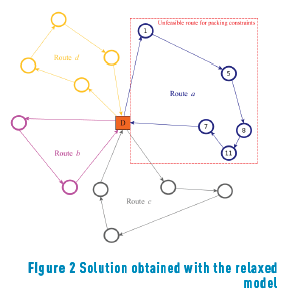A solution for the relaxed MCVRP could consider subtours and also routes that are unfeasible respect to the packing constraints (see Route a in Figure 2). The customers belonging to Route a cannot be packed by the violation the sequential loading constraints, i.e., the route 0 – 1 – 5 – 8 – 11 – 7 – 0 must be eliminated. However, several permutations of Route a (example 0 – 7 – 11 – 8 – 5 – 1 – 0) are examined in order to check feasible solutions for the packing constraints. If any permutation of the route is possible to pack, its edges are considered for the next iterations of the Matheuristic. Therefore, the customers belonging to the unfeasible Route a make up the set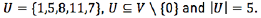Then, according to the Eq. (9), the following cut (10) is applied: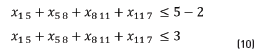Consequently, any subsets of those edges are allowed, but the complete route sequence in the initial order is restricted on the following iterations once the model MCVRP applies the cut (10). This process iterates on feasible solutions for the vehicle routing problem, but infeasible for the three-dimensional container loading problem. The optimal solution is found when the entire load of the routes of the optimal solution for the CVRP is also feasible for the 3D-SLOPP.

4.2. GRASP approach

In this work, we have considered an adapted version of the GRASP (Greedy Randomized Adaptive Search Procedure) algorithm presented in . The proposed approach is based on the representation of maximum spaces, which allows obtaining feasible solutions by the control of the generation and the upgrade of these spaces in the constructive phase. The GRASP satisfies the constraints of the orientation of the boxes, load-bearing strength, the limit of the weight, the stability of the load, and the multi-drop patterns (loading with multiple destinations).

The GRASP algorithm was developed by  to solve difficult combinatorial optimization problems. Different researches show its quality and its robustness . GRASP is an iterative procedure that combines a constructive phase and an improvement phase. In the constructive phase, a solution is built step by step, by adding items. The improvement phase is iterative, greedy, random and adaptive. In the following subsections the constructive phase, the random strategy, the moves developed for the improvement phase, and the diversification process, are described. The proposed search strategy allows randomizing the choice of the type and number of boxes to be located at each maximal space. The improvement phase applies several moves (compression of loading boxes and the refilling process).

4.3. Constructive algorithm

The constructive algorithm is based on the idea proposed by  for the classic container-loading problem. The main difference respect to this algorithm is the consideration of vertical stability, which must be guaranteed by packing patterns with full support. In addition, a special treatment to upgrade structures of the remaining available spaces is considered. This aspect is important due to the management of the empty spaces is not longer trivial by trying to remove some items.

The constructive algorithm is based on the utilization of the maximal spaces. In this case, each selected box is packed in a new space, creating three new maximal spaces (see Figure 3). The constructive algorithm uses an updated list (FS) of the maximal spaces and a list B that contains the boxes of the current customers, which are not ready to be packed. The steps of the constructive algorithm are defined below.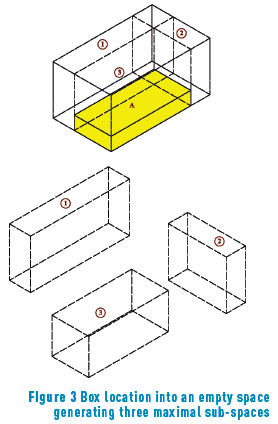Step 0: Initialization of FS . A list FS of empty spaces has been created for locating the selected boxes. Let B = B1, ... , B2, Bn be the list of the set of remaining boxes to be packed for a given customer.

Step 1: Choose a maximal space of FS. Since FS represents the maximum empty spaces with the largest available parallelepipeds to locate the boxes, it is necessary to determine a mechanism of selection of spaces based on some criteria of quality or packing strategies. In this paper, two criteria are proposed: choose the maximum space with the minimum distance to the backside of the container and choose the maximum space with the minimum distance to the roof of the container. In addition, the lower rear corner of the container is selected for the selected space as a reference to locate the boxes in the empty space.

Step 2: Select the boxes to be packed. Once the maximal space FS´ has been selected, it is necessary to consider the sorted list B of the first box ithat fits inside FS´. If there are multiple boxes type i , it is mandatory to generate each of the possible layers. Therefore, the boxes must be packed in arrays of columns or rows by combining the different axes. As in , two criteria are considered to select one of the configurations of boxes:

· Select the layer of boxes that produces the largest increase in the objective function (maximum volume). This is a greedy approach filling the space with the layer of a greater volume of the boxes.

· Select the layer of boxes that best fit in the maximum space. This is a criterion for which the distances between each side of the layer of boxes and each face of the maximum space are computed. The distances of each configuration are ordered in non-decreasing way for selecting the configuration with the lowest distance (which best fits to the space).

Step 3: Update the list FS. The packing process produces new maximal spaces FS´ to be replaced in the list FS, except for the case either the box or the layer fits exactly in the space FS. Moreover, as the maximal spaces are not disjoint, the packed box (or the layer) can be intercepted with other maximal spaces, which could be reduced or eliminated.

The list FSis verified and updated once the new spaces have been added and some existing spaces have been modified. The list B is also updated and the maximal spaces that cannot locate of any of the boxes that still remain to pack must be removed from S. If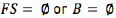, this phase is finished. Otherwise, if there are still boxes to be packed of the current customer, the algorithm must return to step 1.

The list must be verified and the possible options be removed of the list, once the maximum spaces have been added and some existing spaces have been modified.

Step 4: Update the list S for a new customer. When the current customer has been packed, the maximal spaces must be updated depending of the criterion of multi-drop.

· Visible: The maximal spaces that are completely invisible from the door of the container must be removed from the list. In addition, the maximal spaces having a visible and an invisible part must be modified.

· Achievable: The unreachable spaces must be removed or updated.

4.4. Randomization

A layer is built according to the selected criterion (maximum volume or best fit) for each type of box and for each allowed orientation. Each layer is called as: configuration or candidate. When the full range of potential layers is constructed, a restricted list of candidates is considered by selecting one of these layers randomly. We used a Restricted Candidate List (RCL) according to a determined value (this means that the candidates are sorted according to their quality value). If the value of the objective function of the candidate is greater than a threshold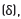the candidate is located in the list RCL. In the process of building layers, we have considered the value C for each candidate, its lowest (Cmin) value and its highest value (Cmax) . The candidate is accepted into the RCL only if it satisfies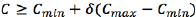. The parameter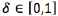controls the size of the list of candidates. If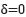, all the configurations are randomly selected and considered to the list. In contrast,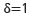indicates a completely greedy selection, because only the best candidate would be the only element in the list and it always be chosen. For values of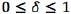, the number of configurations in the list is not predefined, depending on the relative values of the candidates. In this case,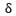takes randomly one value of 11 possibilities 0, 0.1, …, 0.9 and 1. This value is selected depending on its performance in previous iterations. Indeed, if the value ofhas improved the current solution previously, the probability of its selection is increased and decreased otherwise.

4.5. Improvement

The improvement movement consists of eliminating the last k% boxes packed in the complete solution. We choose the value k at random from the interval (30, 90) as in . The removed items plus the items that were left unpacked in the solution are then packed again using the deterministic constructive procedure guide by the objective function of Best-Volume. In this call of the deterministic algorithm, we can use the objective function Best-Volume. We consider that a solution has improved if the total volume of the packed boxes has increased.

The improvement phase is only called if the solution of the constructive phase is considered to be promising, that is, if it is considered a good starting point for improving on the best known solution. Therefore, we only consider those solutions that are above a certain threshold. At the beginning, the threshold takes the value of the first solution of the constructive algorithm. Then, if at an iteration the solution value is greater than the threshold, we update this threshold to this value and go to the improvement phase. If the solution value is lower than the threshold, the solution is not improved and the reject counter (niter) is increased. When the number of rejected solutions is greater than a value maxFilter, the threshold is decreased according to the expression:

threshold = threshold -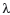(1+threshold) whereis set at 0.2 (as in ), and maxFilter = 50% total iterations.

Figure 4 shows the GRASP approach used to solve the packing subproblem of the proposed algorithm. The GRASP Algorithm begins by selecting one of the empty spaces ( FSi), and then the list of layers of boxes (Cs) that fit in the space FSi is generated. Then, the list C is reduced to the Restricted Candidate List (RCL). One element is randomly selected of the list Cs. The layer Cis located generating the pattern P and forcing to update the lists of maximum spaces and the lists of the remaining boxes (FS and B,respectively). When all the boxes demanded by a customer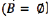are assigned, the objective function is analyzed determining its quality. If it has good quality, the best solution found so far is updated. If there are still empty remaining spaces and there are customers for packing, then the maximum space list must be updated by changing customers (it should be necessary to eliminate the spaces that violate the multi-drop constraints). Finally, if there are no empty spaces, the process finishes.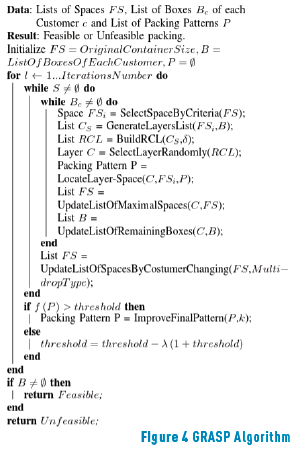5. Computational results

We have considered the classical set of benchmark instances (27 instances) to validate the performance of the proposed methodology. The proposed matheuristic has been compared with [15, 17-19, 24, 29]. Several best known results have been improved. The computing time of the proposed methodology is quite high compared to the published approaches. The benchmark set for the 3L-CVRP has been taken from the library published in http://or.dei.unibo.it/instances/three-dimensional-capacitated-vehicle-routing-problem-3l-cvrp.

Table 1 shows the total cost of the performed routes to deliver all the boxes for the customers (objective function of 3L-CVRP). The Figure 5 shows the routes obtained for the proposed algorithm. Figures 6-9 show the packing patterns corresponding to the routes of the solution for Instance 1. As is shown, the packing patterns satisfy the constraints of static stability, brittleness of the boxes and unloading without rearrangement (multi-drop or LIFO policy).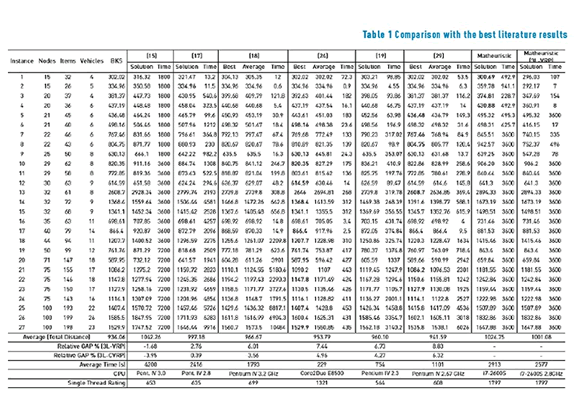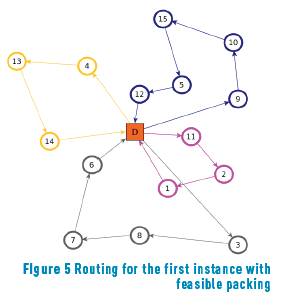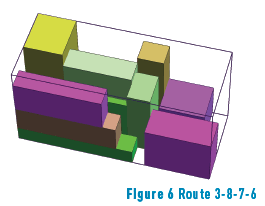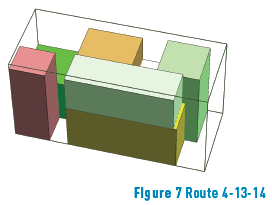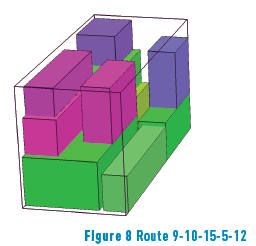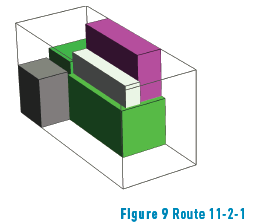The proposed methodology outperforms the quality of the solution found by the previous published algorithms presented in the literature. The computational times are high due to the use of exact method for the vehicle routing problem. The solutions obtained for the integrated 3L-CVRP problem by including packing constraints, make difficult the solution of the generation of routes to deliver products to the customers. The Figure 6 shows the performed routes for Instance 1. Note that some routes are not convex envelopes as in the traditional CVRP (see route located to the right in Figure 4). In addition, the performed routes clearly indicate a worse objective function for the CVRP. Indeed, the CVRP could be considered as a lower bound for the vehicle routing problem of the 3L-CVRP.

For the case of transportation of one type of structure of the boxes, the obtained results of the proposed approach respect to the packing constraints (see column Matheuristic (3L-VRP) in Table 1) are aligned with the conclusions of . Indeed, the obtained solutions for the benchmarking set are at least of the same quality than the case where all the type of density of boxes for each customer (see column Matheuristic of Table 1). In this paper, the proposed algorithm considers infeasible solutions, iteratively by worsening the transportation costs until reaching a feasible solution of minimum cost packing the demand of customers of the vehicles.

6. Concluding remarks and future research

In this paper, a successful matheuristic algorithm has been proposed for solving the 3L-CVRP. The hybrid methodology decomposes the 3L-CVRP into two subproblems: the Capacitated Vehicle Routing Problem (CVRP) and the Three-dimensional Container Loading Problem (3D-SLOPP). The proposed approach combines a branch-and-cut algorithm for solving the vehicle routing problem (CVRP) with a GRASP approach in order to find a feasible solution for the 3D-SLOPP. The proposed algorithm has been compared with [15, 17-19, 24, 29] on the classical set of benchmark instances proposed for the 3L-CVRP. The results show the effectiveness of the proposed approach (some new best known solutions are found).

For future research, we will consider other mathematical formulations (i.e. three index mathematical formulation) that can be decomposed by exploiting the benefits of a brach-and-cut technique or a generation of columns. This consideration has advantages for solving the CVRP due to provides the control of the routes individually. In addition, it is possible to remove easily the capacity and subtour elimination constraints. However, it is necessary to make a careful treatment of this model because it implies an increase remarkable of the number of variables.

7. References

1. P. Augerat et al., "Computational results with a branch and cut code for the capacitated vehicle routing problem", Université Joseph Fourier, Grenoble, France, Tech. Rep. 949-M, 1995.         [ Links ]

2. P. Toth and D. Vigo, The Vehicle Routing Problem, Monographs on Discrete Mathematics and Applications, Philadelphia, USA, 2002.         [ Links ]

3. B. Golden, S. Raghavan and E. Wasil, The Vehicle Routing Problem: Latest Advances and New Challenges. USA: Springer, 2008.         [ Links ]

4. R. Baldacci, P. Toth and D. Vigo, "Exact algorithms for routing problems under vehicle capacity constraints", Annals of Operations Research, vol. 175, no. 1, pp. 213-245, 2010.         [ Links ]

5. V. Schmid, K. Doerner and G. Laporte, "Rich routing problems arising in supply chain management", European Journal of Operational Research, vol. 224, no. 3, pp. 435-448, 2013.         [ Links ]

6. A. Lodi, S. Martello and M. Monaci, "Two-dimensional packing problems: A survey", European Journal of Operational Research, vol. 141, no. 2, pp. 241-252, 2002.         [ Links ]

7. V. Paschos, Paradigms of Combinatorial Optimization: Problems and New Approaches. John Wiley & Sons, 2013.         [ Links ]

8. G. Wascher, H. Haubner and H. Shchumann, "An improved typology of cutting and packing problems", European Journal of Operational Research, vol. 183, no. 3, pp. 1109-1130, 2007.         [ Links ]

9. E. Coffman, J. Csirik, G. Galambos, S. Martello and D. Vigo, "Bin Packing Approximation Algorithms: Survey and Classification", in Handbook of Combinatorial Optimization, 2nd ed., P. Pardalos, D. Du and R. Graham (eds): New York, USA: Springer, 2013, pp. 455-531.         [ Links ]

10. M. Alonso, R. Alvarez, J. Tamarit and F. Parreño, "A reactive GRASP algorithm for the container loading problem with load-bearing constraints", European Journal of Industrial Engineering, vol. 8, no. 5, pp. 669-694, 2014.         [ Links ]

11. D. Álvarez, F. Parreño, R. Álvarez and F. Parreno, "A grasp algorithm for the container loading problem with multi-drop constraints", Pesqui. Oper., vol. 35, no. 1, pp. 1-24, 2015.         [ Links ]

12. L. Junqueira, J. Oliveira, M. Carravilla and R. Morabito, "An optimization model for the vehicle routing problem with practical three-dimensional loading constraints", International Transactions in Operational Research, vol. 20, no. 5, pp. 645-666, 2013.         [ Links ]

13. A. Moura and J. Oliveira, "An integrated approach to vehicle routing and container loading problems", OR Spectrum, vol. 31, no. 4, pp. 775-800, 2009.         [ Links ]

14. A. Moura, "A multi-objective genetic algorithm for the vehicle routing with time windows and loading problem", in Intelligent Decision Support, 1st ed., A. Bortfeldt, J. Homberger, H. Kopfer, G. Pankratz and R. Strangmeier (eds). Frankfurt, Germany: Springer, 2008, pp. 187-201.         [ Links ]

15. M. Gendreau, M. Iori, G. Laporte and S. Martello, "A tabu search algorithm for a routing and container loading problem", Transportation Science, vol. 40, no. 3, pp. 342-350, 2006.         [ Links ]

16. Y. Tao and F. Wang, "A new packing heuristic based algorithm for vehicle routing problem with three-dimensional loading constraints", in Conference on Automation Science and Engineering (CASE), Toronto, Canada, 2010, pp. 972-977.         [ Links ]

17. C. Tarantilis, E. Zachariadis, and C. Kiranoudis, "A hybrid metaheuristic algorithm for the integrated vehicle routing and three-dimensional container-loading problem", IEEE Transactions on Intelligent Transportation Systems, vol. 10, no. 2, pp. 255-271, 2009.         [ Links ]

18. G. Fuellerer, K. Doerner, R. Hartl and M. Iori, "Metaheuristics for vehicle routing problems with three-dimensional loading constraints", European Journal of Operational Research, vol. 201, no. 3, pp. 751-759, 2010.         [ Links ]

19. Q. Ruan, Z. Zhang, L. Miao and H. Shen, "A hybrid approach for the vehicle routing problem with three-dimensional loading constraints", Computers & Operations Research, vol. 40, no. 6, pp. 1579-1589, 2013.         [ Links ]

20. L. Miao, Q. Ruan, K. Woghiren and Q. Ruo, "A hybrid genetic algorithm for the vehicle routing problem with three-dimensional loading constraints", RAIRO - Operations Research, vol. 46, no. 1, pp. 63-82, 2012.         [ Links ]

21. W. Zhu, H. Qin, A. Lim and L. Wang, "A two-stage tabu search algorithm with enhanced packing heuristics for the 3l-cvrp and m3l-cvrp", Computers & Operations Research, vol. 39, no. 9, pp. 2178-2195, 2012.         [ Links ]

22. G. Koloch and B. Kaminski, "Nested vs. joint optimization of vehicle routing problems with three-dimensional loading constraints", Engineering Letters, vol. 18, no. 2, pp. 193-198, 2010.         [ Links ]

23. A. Bortfeldt and J. Homberger, "Packing first, routing second - a heuristic for the vehicle routing and loading problem", Computers & Operations Research, vol. 40, no. 3, pp. 873-885, 2013.         [ Links ]

24. A. Bortfeldt, "A hybrid algorithm for the capacitated vehicle routing problem with three-dimensional loading constraints", Computers & Operations Research, vol. 39, no. 9, pp. 2248-2257, 2012.         [ Links ]

25. T. Feo and M. Resende, "A probabilistic heuristic for a computationally difficult set covering problem", Operations Research Letters, vol. 8, no. 2, pp. 67-71, 1989.         [ Links ]

26. M. Resende and C. Ribeiro, "Greedy Randomized Adaptive Search Procedures", in Handbook of Metaheuristics, 1st ed., F. Glover and G. Kochenberger (eds). Norwell, USA: Kluwer Academic Publishers, 2003, pp. 219-249.         [ Links ]

27. F. Parreño, R. Álvarez, J. Tamarit and J. Oliveira, "Neighborhood structures or the container loading problem: A VNS implementation", Journal of Heuristics, vol. 16, no. 1, pp. 1-22, 2010.         [ Links ]

28. R. Marti and J. Moreno, "Métodos multiarranque", Inteligencia Artificial, vol. 7, no. 19, pp. 49-60, 2003.         [ Links ]

29. Y. Tao and F. Wang, "An effective tabu search approach with improved loading algorithms for the 3l-cvrp," Computers Operations Research, vol. 55, pp. 127-140, 2015.         [ Links ]